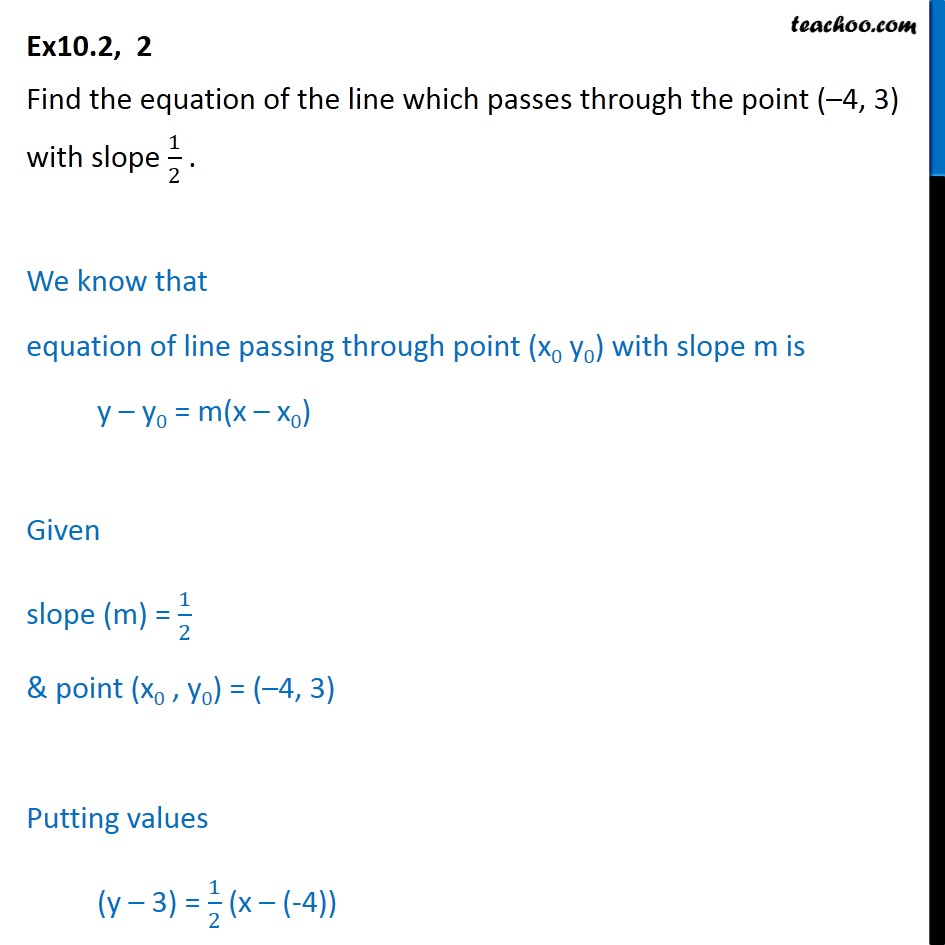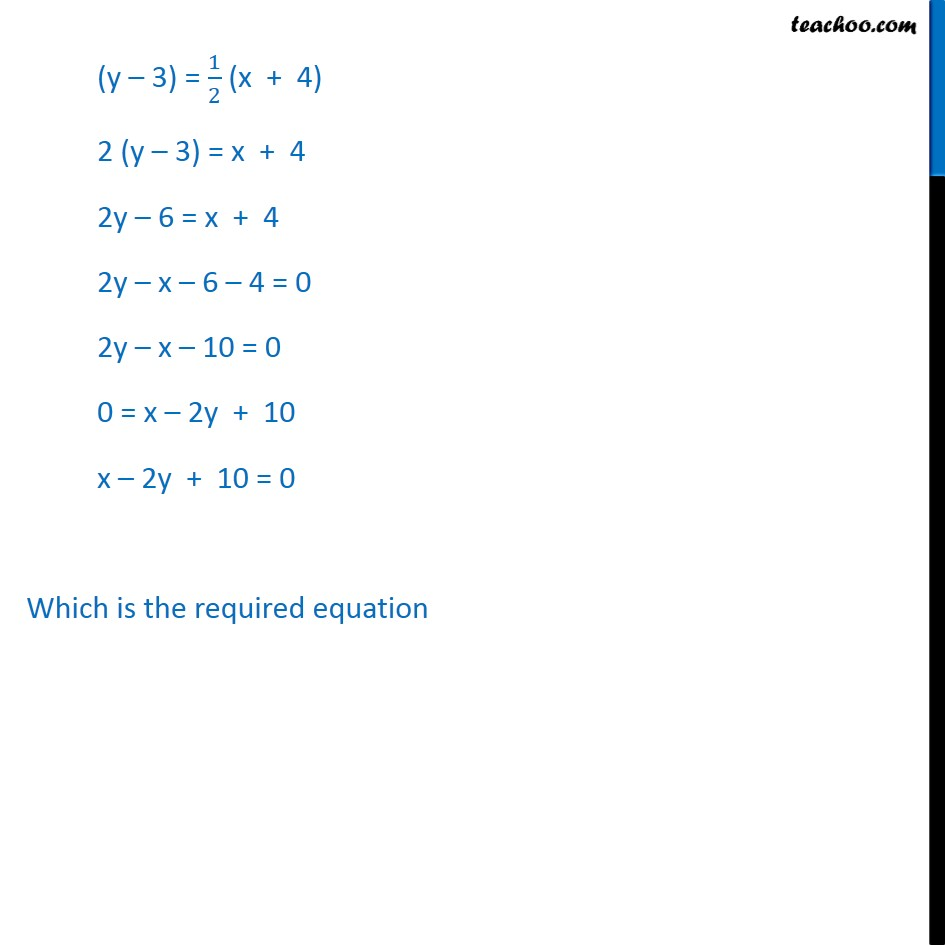Ex 9.2

Chapter 9 Class 11 Straight Lines
Serial order wiseLearn in your speed, with individual attention - Teachoo Maths 1-on-1 Class

### Transcript

Ex10.2, 2 Find the equation of the line which passes through the point ( 4, 3) with slope 1/2 . We know that equation of line passing through point (x0 y0) with slope m is y y0 = m(x x0) Given slope (m) = 1/2 & point (x0 , y0) = ( 4, 3) Putting values (y 3) = 1/2 (x (-4)) (y 3) = 1/2 (x + 4) 2 (y 3) = x + 4 2y 6 = x + 4 2y x 6 4 = 0 2y x 10 = 0 0 = x 2y + 10 x 2y + 10 = 0 Which is the required equation